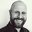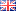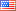Question-and-Answer Resource for the Building Energy Modeling Community
Get s tarted with the Help page

# Revision history [back]

### How can I simulate a CoolingCoilDX with a high air flow (rated air volume flow rate)?

I'm simulating a DataCenter equipped with CRACs. These devices have a very high rated air volume flow rate. For the equipment I'm using the ratio is 0.00006822 m³/s per W (0.2456 m³/h per W or 865 m³/h per TR).

The problem is that the Coil equipment: Cooling: DX: Single: Speed accepts a maximum 0.00006041 m³/s per W and when I put a higher flow relation to this, the error of EnergyPlus reporting me that the flow ratio by load is higher than the maximum acceptable.

* Severe * Sizing: Coil:Cooling:DX:SingleSpeed "CRAC - DATA CENTER": Rated air volume flow rate per watt of rated total cooling capacity is out of range. * ~~~ * Min Rated Vol Flow Per Watt=[4.027E-005], Rated Vol Flow Per Watt=[6.821E-005], Max Rated Vol Flow Per Watt=[6.041E-005]. See Input Output Reference Manual for valid range.

Is there any other component in the EnergyPlus that properly represents a CRAC and supports a greater rated air volume flow rate ratio.2 No.2 RevisionJamie Bull4972 ●6 ●23 http://oco-carbon.com/

### How can I simulate a CoolingCoilDX with a high air flow (rated air volume flow rate)?

I'm simulating a DataCenter equipped with CRACs. These devices have a very high rated air volume flow rate. For the equipment I'm using the ratio is 0.00006822 m³/s per W (0.2456 m³/h per W or 865 m³/h per TR).

The problem is that the Coil equipment: Cooling: DX: Single: Speed equipment Coil:Cooling:DX:SingleSpeed accepts a maximum 0.00006041 m³/s per W and when I put a higher flow relation to than this, the EnergyPlus reports an error of EnergyPlus reporting me that the flow ratio by load is higher than the maximum acceptable.

*

** Severe  * ** Sizing: Coil:Cooling:DX:SingleSpeed "CRAC - DATA CENTER": Rated air volume flow rate per watt of rated total cooling capacity is out of range.
* **   ~~~   * ** Min Rated Vol Flow Per Watt=[4.027E-005], Rated Vol Flow Per Watt=[6.821E-005], Max Rated Vol Flow Per Watt=[6.041E-005]. See Input Output Reference Manual for valid range.range.


Is there any other component in the EnergyPlus that properly represents a CRAC and supports a greater rated air volume flow rate ratio.ratio?3 retagged__AmirRoth__4388 ●5 ●16 http://bleedinggreenna...

### How can I simulate a CoolingCoilDX with a high air flow (rated air volume flow rate)?

I'm simulating a DataCenter equipped with CRACs. These devices have a very high rated air volume flow rate. For the equipment I'm using the ratio is 0.00006822 m³/s per W (0.2456 m³/h per W or 865 m³/h per TR).

The problem is that the equipment Coil:Cooling:DX:SingleSpeed accepts a maximum 0.00006041 m³/s per W and when I put a higher flow relation than this, EnergyPlus reports an error that the flow ratio by load is higher than the maximum acceptable.

** Severe  ** Sizing: Coil:Cooling:DX:SingleSpeed "CRAC - DATA CENTER": Rated air volume flow rate per watt of rated total cooling capacity is out of range.
**   ~~~   ** Min Rated Vol Flow Per Watt=[4.027E-005], Rated Vol Flow Per Watt=[6.821E-005], Max Rated Vol Flow Per Watt=[6.041E-005]. See Input Output Reference Manual for valid range.


Is there any other component in EnergyPlus that properly represents a CRAC and supports a greater rated air volume flow rate ratio?4 retagged__AmirRoth__4388 ●5 ●16 http://bleedinggreenna...

### How can I simulate a CoolingCoilDX with a high air flow (rated air volume flow rate)?

I'm simulating a DataCenter equipped with CRACs. These devices have a very high rated air volume flow rate. For the equipment I'm using the ratio is 0.00006822 m³/s per W (0.2456 m³/h per W or 865 m³/h per TR).

The problem is that the equipment Coil:Cooling:DX:SingleSpeed accepts a maximum 0.00006041 m³/s per W and when I put a higher flow relation than this, EnergyPlus reports an error that the flow ratio by load is higher than the maximum acceptable.

** Severe  ** Sizing: Coil:Cooling:DX:SingleSpeed "CRAC - DATA CENTER": Rated air volume flow rate per watt of rated total cooling capacity is out of range.
**   ~~~   ** Min Rated Vol Flow Per Watt=[4.027E-005], Rated Vol Flow Per Watt=[6.821E-005], Max Rated Vol Flow Per Watt=[6.041E-005]. See Input Output Reference Manual for valid range.


Is there any other component in EnergyPlus that properly represents a CRAC and supports a greater rated air volume flow rate ratio?